• 当需要判断两种现象或者变量之间是否存在依赖关系，若存在，是什么依赖关系以及依赖程度时，我们就需要对着两种想想或变量进行相关分析。本文总结了有关相关系数分析方法的示意图，如图1所示： 图1 相关分析方法分类...


当需要判断两种现象或者变量之间是否存在依赖关系，若存在，是什么依赖关系以及依赖程度时，我们就需要对着两种想想或变量进行相关分析。本文总结了有关相关系数分析方法的示意图，如图1所示：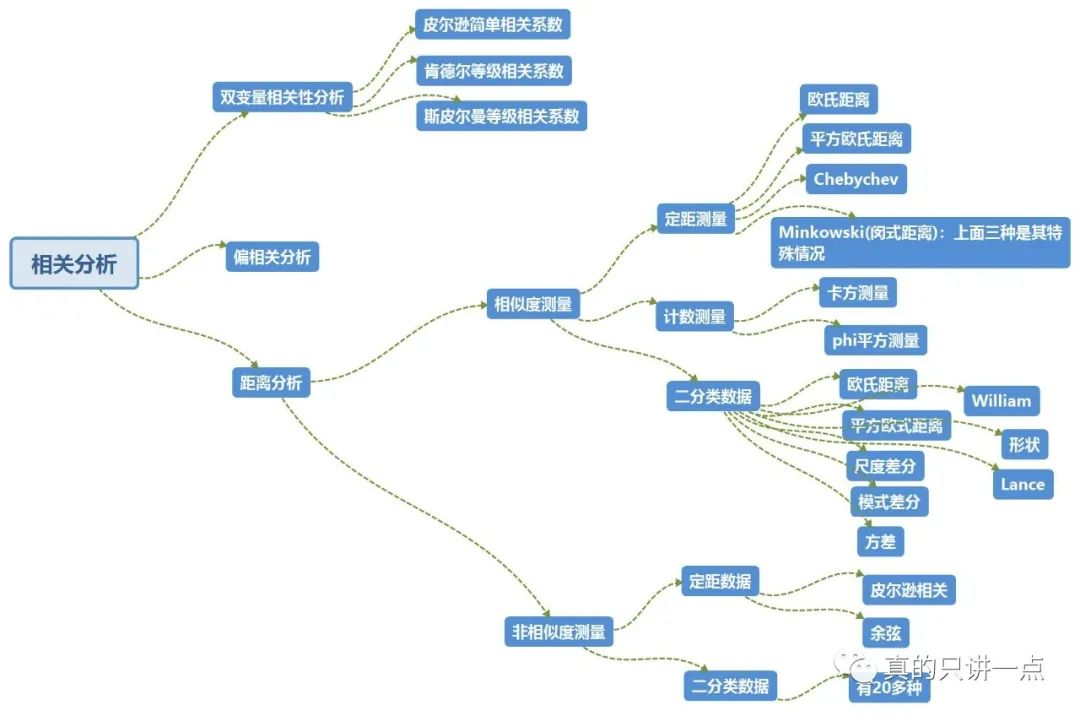图1 相关分析方法分类示意图图1已经给出了相关分析的分类，下面讨论各种分类方法的使用场景以及本人认为比较有趣的相关理论。1 双变量相关分析使用场景：只有两个变量方法：计算相关系数公式：a)皮尔逊简单相关系数两个变量之间的协方差和标准差的商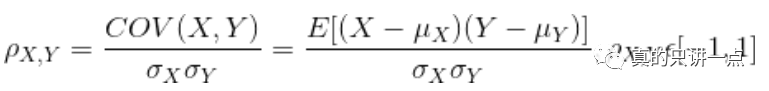当皮尔逊相关系数越小，两个变量的向相关性越低b)肯德尔等级相关系数该系数有三种情况，又不常用，在这不予解释c)斯皮尔曼等级相关系数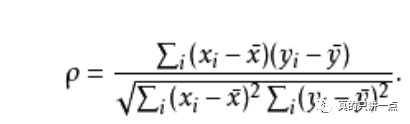2 偏相关分析使用场景：数据中包含多个变量，多个变量之间可能有相互联系，但是我们只想对其中的两个变量进行分析。在这种情况下，就需要用偏相关分析排除其他变量对着两个观察变量的影响。3 距离分析使用场景：数据包含多个变量，将这些变量按照一定的标准(距离)分类，进行聚类分析从图1中可以看出，距离分析包含许多方法，这里面有个Minkowski方法，即我们常说的闵式距离，是由爱因斯坦在瑞士苏黎世联邦科技大学的数学老师最先提出，表达式为：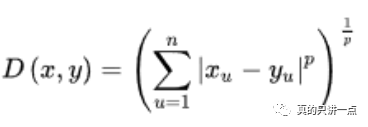这里的参数p是个定值，当它取不同的值时，就是图1中定距测量的其他方法：a) p=1,D(x,y)表示绝对距离，也叫曼哈顿距离(Manhattan distance)、出租汽车距离或街区距离(city block distance)：是计算两个点之间存在障碍物，不能直线到达，拐过一个直角的最小两条直角边的距离。b) p=2,D(x,y)表示欧氏距离：两点之间的直线距离。c) p趋于无穷时,D(x,y)表示切比雪夫距离，也称棋盘距离：两点之间各坐标数值差的绝对值的最大值。以(x1,y1)和(x2,y2)二点为例，其切比雪夫距离为max(|x2-x1|,|y2-y1|)。总结：本文总结了相关分析的使用场景和各种相关分析方法及其具体方法，为以后再用SPSS及其他需求来处理数据时需要用到的分析方法提供快速检索。

展开全文• 方差分析与回归、相关分析：回归与相关处理的是两个数值变量的问题，相应的散点在 xxx 轴上具有顺序（从小到大），而方差分析的数据在 xxx 轴上可以任意交换位置。 1.4 数学模型 1、定义 响应变量（因变量
方差分析
一、基本概念
1.1 定义

研究一个（或多个）分类自变量如何影响一个数值因变量的统计分析方法

1.2 目的

判断某些因素对于我们感兴趣的因变量是否具有“显著”的影响
如果因素间有交互效应，寻找最佳搭配方案

1.3 特点

方差分析与一般的假设检验：方差分析处理的是多个均值的情况
方差分析与回归、相关分析：回归与相关处理的是两个数值变量的问题，相应的散点在 $x$ 轴上具有顺序（从小到大），而方差分析的数据在 $x$ 轴上可以任意交换位置。

1.4 数学模型
1、定义

响应变量（因变量）：进行随机试验所考察的数值指标
因素或因子（自变量）：影响因变量的各不同分类原因
水平：各个因素所构成的组或者类型

2、例子：考察小麦产量（$y$）对于品种和施肥量的关系（两个不同的小麦品种，三个不同的施肥等级）

品种是否对产量有影响 $\Leftrightarrow H_{01}: \alpha_1 = \alpha_2$
施肥量是否对产量有影响 $\Leftrightarrow H_{02}: \beta_1 = \beta_2 = \beta_3$
$Y = X\beta + \varepsilon$
$\begin{cases} y_{11} = \theta_0 + \alpha_1 + \beta_1 + \varepsilon_{11} \\ y_{12} = \theta_0 + \alpha_1 + \beta_2 + \varepsilon_{12} \\ y_{13} = \theta_0 + \alpha_1 + \beta_3 + \varepsilon_{13} \\ y_{21} = \theta_0 + \alpha_2 + \beta_1 + \varepsilon_{21} \\ y_{22} = \theta_0 + \alpha_2 + \beta_2 + \varepsilon_{22} \\ y_{23} = \theta_0 + \alpha_2 + \beta_3 + \varepsilon_{23} \end{cases}$

二、单因素方差分析
2.1 数据的结构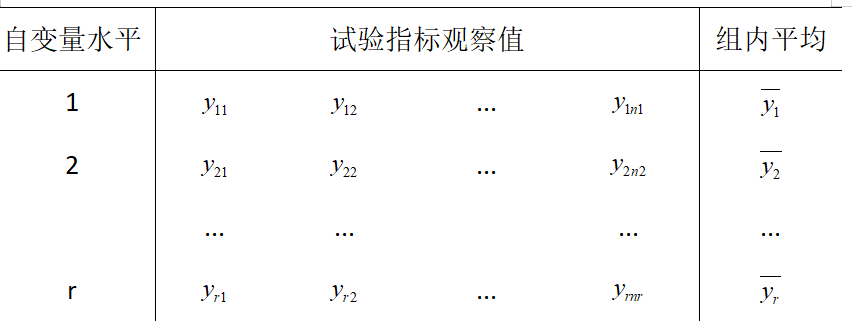$y_{ij} = \beta_i + \varepsilon_{ij} ,\,\,\,\,\,\, 1 \le j \le n_i 、1 \le i \le r$
主要任务：

检验假设： $H_0: \beta_1 = \beta_2 = ... = \beta_r$
作出未知参数：$\beta_1, \beta_2, ... , \beta_r$ 以及 $\sigma^2$ 估计

2.2 因子效应与误差方差的估计

按照模型的假定，因变量的观察值来自 $r$ 个不同的正态总体
$y_{11}, ..., y_{1n1}$ 来自总体 $N(\beta_1, \sigma^2)$
未知参数 $\beta_1, \beta_2, ... , \beta_r$ 的估计就采用各个总体的样本均值

2.3 相关定义

因素各水平效应的估计采用各个组内平均
$\hat{\beta_i} = \overline{y_i} = \frac{1}{n_i} \sum_{j=1}^{n_i} y_{ij} \Leftrightarrow N(\beta_i, \frac{\sigma^2}{n_i}), 1 \le i \le r$
误差方差 $\sigma^2$ 的估计利用残差平方和
$\hat{\sigma}^2 = \frac{RSS}{n-r} = \frac{1}{n-r} \sum_{i=1}^r \sum_{j=1}^{n_s} (y_{ij} - \overline{y_i})^2$
$\frac{(n-r)\hat{\sigma}^2}{\sigma^2} \Leftrightarrow \chi^2(n-r)$
$\hat{\beta_1}, \hat{\beta_2}, ... , \hat{\beta_r}, \hat{\sigma}^2$ 之间相互独立

2.4 方差分析平方和分解公式

总平方和：表示因变量总的变化。（因子不同的水平，随机误差）
$TSS = \sum_{i=1}^{r} \sum_{j=1}^{n_i}(y_{ij} - \overline{y})^2$
自变量平方和：表示自变量在因变量的变化中所占的份额
$CSS = \sum_{i=1}^{r} n_i (\overline{y_i} - \overline{y})^2$
残差平方和：表示由其它原因引起的因变量变化
$RSS = \sum_{i=1}^{r} \sum_{j=1}^{n_i}(y_{ij} - \overline{y_i})^2$
$TSS = CSS + RSS$

2.5 单因素方差分析的检验

如果零假设 $H_0: \beta_1 = \beta_2 = ... = \beta_r$ 成立，则
$\frac{CSS}{\sigma^2} \Leftrightarrow \chi^2(r-1)$
检验统计量
$F比 = \frac{n-r}{r-1} \frac{CSS}{RSS} \Leftrightarrow F(r-1, n-r)$
拒绝域
$F \ge F_\alpha(r-1, n-r)$

2.6 单因素方差分析表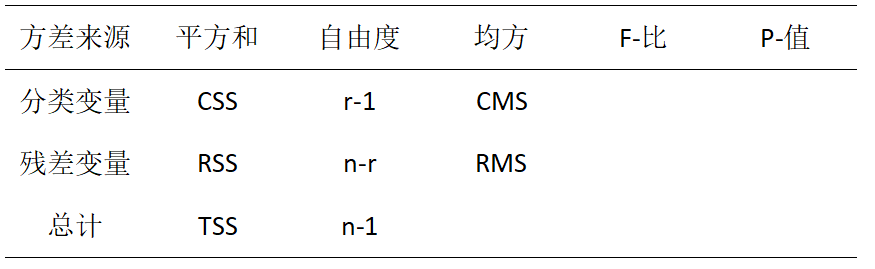$CMS = \frac{CSS}{r-1}, RMS = \frac{RSS}{n-r}, F-比 = \frac{CMS}{RMS}$
2.7 变量关系的强度
$R^2 = \frac{自变量平方和}{总平方和} = \frac{CSS}{TSS}$
三、双因素方差分析
3.1 数据的结构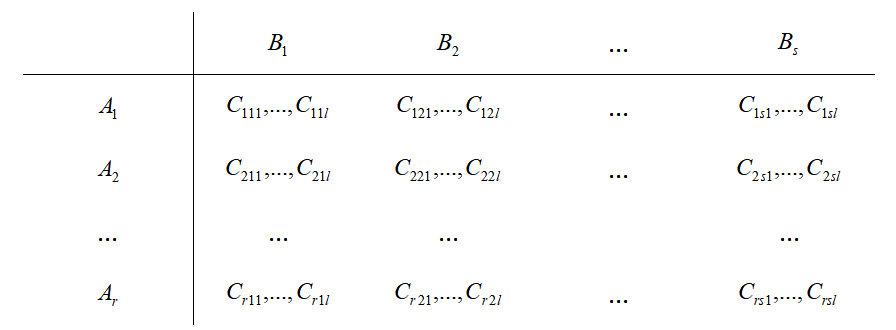$y_{ijk} = \mu + \alpha_i + \beta_j + \gamma_{ij} + \varepsilon_{ijk}, 1 \le i \le r, 1 \le j \le s, 1 \le k \le l, \varepsilon_{ijk} \Leftrightarrow N(0, \sigma^2)$
主要任务：

因子的主效应是否显著，即检验： $H_{01}: \alpha_1 = \alpha_2 = ... = \alpha_r$，以及 $H_{02}: \beta_1 = \beta_2 = ... = \beta_s$
交互效应是否显著：$H_{03}: \gamma_{11} = \gamma_{12} = ... = \gamma_{rs}$
如果拒绝了 $H_{03}$ ，还应该寻找最佳搭配。

3.2 相关定义

总平均
$\overline{y} = \frac{1}{rsl} \sum_{i=1}^r \sum_{j=1}^s \sum_{k=1}^l y_{ijk}$
误差平均
$\overline{y_{ij·}} = \frac{1}{l} \sum_{k=1}^l y_{ijk}$
$A$ 因素平均
$\overline{y_{i··}} = \frac{1}{s} \sum_{j=1}^s \overline{y_{ij·}}$
$B$ 因素平均
$\overline{y_{·j·}} = \frac{1}{r} \sum_{i=1}^r \overline{y_{ij·}}$

3.3 方差分析平方和分解公式

总平方和
$TSS = \sum_{i=1}^r \sum_{j=1}^s \sum_{k=1}^l (y_{ijk} - \overline{y})^2$
$A$ 因子主效应平方和
$SSA = sl \sum_{i=1}^r (\overline{y}_{i··} - \overline{y})^2$
$B$ 因子主效应平方和
$SSB = rl \sum_{j=1}^s (\overline{y}_{·j·} - \overline{y})^2$
交互效应平方和
$SSAB = l \sum_{i=1}^r \sum_{j=1}^s (\overline{y}_{ij·} - \overline{y}_{i··} - \overline{y}_{·j·} + \overline{y})^2$
随机误差平方和
$RSS = \sum_{i=1}^r \sum_{j=1}^s \sum_{k=1}^l (y_{ijk} - \overline{y}_{ij·})^2$
$TSS = SSA + SSB +SSAB + RSS$

3.4 单因素方差分析的检验

$\frac{RSS}{\sigma^2}$~$\chi^2(rs(l-1))$
当 $H_{01}$ 成立时，$\frac{SSA}{\sigma^2}$~$\chi^2(r-1)$
当 $H_{02}$ 成立时，$\frac{SSB}{\sigma^2}$~$\chi^2(s-1)$
当 $H_{03}$ 成立时，$\frac{SSAB}{\sigma^2}$~$\chi^2((r-1)(s-1))$
对于零假设 $H_{01}: \alpha_1 = \alpha_2 = ... = \alpha_r$，相应的统计量及拒绝域为
$F_A = \frac {rs(l-1)} {r-1} \frac{SSA}{RSS} \Leftrightarrow F(r-1, rs(l-1))$
$\{ F_A \ge F_\alpha(r-1, rs(l-1)) \}$
对于零假设 $H_{02}: \beta_1 = \beta_2 = ... = \beta_s$，相应的统计量及拒绝域为
$F_B = \frac {rs(l-1)} {s-1} \frac{SSB}{RSS} \Leftrightarrow F(s-1, rs(l-1))$
$\{ F_B \ge F_\alpha(s-1, rs(l-1)) \}$
对于零假设 $H_{03}: \gamma_{11} = \gamma_{12} = ... = \gamma_{rs}$，相应的统计量及拒绝域为
$F_{AB} = \frac {rs(l-1)} {(r-1)(s-1)} \frac{SSAB}{RSS} \Leftrightarrow F((r-1)(s-1), rs(l-1))$
$\{ F_{AB} \ge F_\alpha((r-1)(s-1), rs(l-1)) \}$

3.5 双因素方差分析表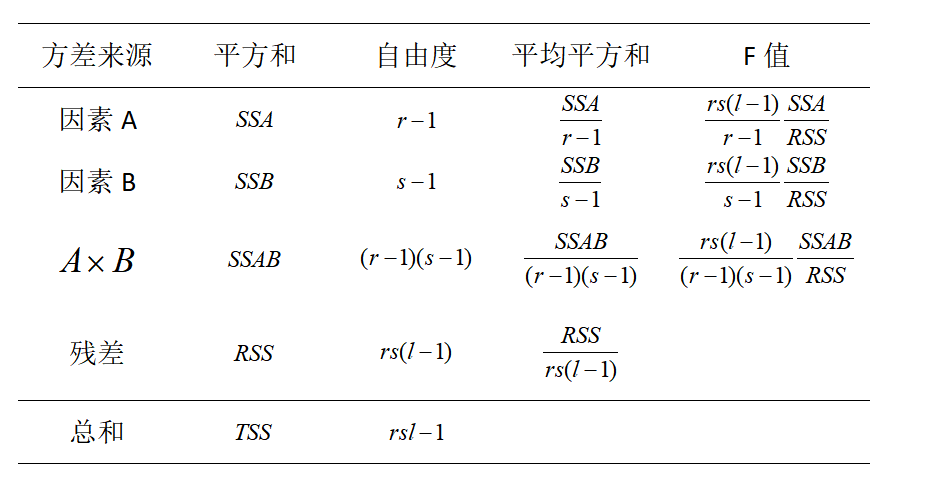展开全文• 本文的研究主要针对环境智能中的两个研究重点：行为识别和目标定位。对 于行为识别，介绍了基于环境变量的行为识别、人物交互式行为识别和穿戴式行 为识别三种方法，并着重分析了穿戴式行为识别方法。穿戴式行为识别...
• 判断文件或目录是否存在 如何读写文件 7.Java多态的实现（继承、重载、覆盖） 8.编码转换，怎样实现将GB2312编码的字符串转换为ISO-8859-1编码的字符串。 9.Java中访问数据库的步骤，Statement和...JAVA
• 这份列表非常有意思：该列表有两个条目，但并没有更多的信息。人名只有首字母，没有 全称；只有房间号，但没有街道名；只有电话号码的一部分，却没有完整的号码。 让我们看另外一个例子，如表1 - 2所示。 表1-2 电话...php
• 5\添加变量方法:进入管理界面-语言修改-$addVariable变量里定义变量名称,可以添加无限个变量,在文章管理里会自动要求设定变量值的. 6\调用变量方法:在templates文件夹下对应的语言的模板里设置.以{$变量名}格式调用....
• 3，不使用中间变量交换两个变量的值 4,python四大内置高阶函数 5，python带颜色输出 6,python *args,**kargs用法 7，python常用模块 8，python多线程 9,python发送邮件 10,python操作图像 11，python的replace()方法...自动化测试面试题
• ## 疯狂ios讲义源代码

千次下载 热门讨论 2014-01-02 09:12:50
5.8.3 判断指针变量的实际类型 197 5.9 小结 198 第6章　面向对象（下） 199 6.1 objective-c的包装类 200 6.1.1 它们不是包装类 200 6.1.2 nsvalue和nsnumber 201 6.2 处理对象 202 6.2.1 打印对象和...
• 在某些应用中，对线性链表中的每个结点设置两个指针，一个称为左指针，用以指向其前件结点；另一个称为右指针，用以指向其后件结点。这样的表称为双向链表。 在线性链表中，各数据元素结点的存储空间可以是不连续的...算法
• 　使用表驱动方法两个问题 　18.2 直接访问表 　示例：一个月中的天数（Days-in-Month） 　示例：保险费率 　例子：灵活的消息格式（Flexible-Message-Format） 　构造查询键值 　18.3 索引表访问（Indexed Access...
• 2.8.2　c#基础语言中是否有全局变量　52 2.8.3　c#字符类型中的string和sting区别　52 2.9　精彩回顾　52 第3章　峰回路转——流程语句控制和数组 ]　10编程技术要点 ]　6编程技巧说明 ]　12编程注意...
• 2.8.2　c#基础语言中是否有全局变量　52 2.8.3　c#字符类型中的string和sting区别　52 2.9　精彩回顾　52 第3章　峰回路转——流程语句控制和数组 ]　10编程技术要点 ]　6编程技巧说明 ]　12编程注意...
• 12.2.2 判断文件是否存在 224 12.2.3 复制文件 225 12.2.4 删除文件 226 12.2.5 文件的加密和解密 227 12.2.6 文件读取 228 12.3 文件夹类Directory 229 12.3.1 创建文件夹 229 12.3.2 删除文件夹 230 12.3.3 获取...
• ## 轻松学C#(图解版)

热门讨论 2014-04-27 19:50:54
12.2.2 判断文件是否存在 224 12.2.3 复制文件 225 12.2.4 删除文件 226 12.2.5 文件的加密和解密 227 12.2.6 文件读取 228 12.3 文件夹类Directory 229 12.3.1 创建文件夹 229 12.3.2 删除文件夹 230 12.3.3 获取...
• ## C++程序员面试宝典

热门讨论 2013-04-01 13:36:19
面试题85 是否可以确定指针指向一对象 90 面试题86 如何使用指针操作数组 90 面试题87 const对象的指针和const指针的区别 91 面试题88 数组指针与指针数组的区别 92 8.2 函数指针 93 面试题89 什么是函数指针？...C++面试宝典
• 实例159　判断变量是否被初始化 实例160　获取文件创建或修改日期 实例161　自定义错误号 实例162　获取当前打开窗口数量 实例163　随机获取姓名 实例164　防止连续出现多空格 实例165　给程序添加运行参数 实例...